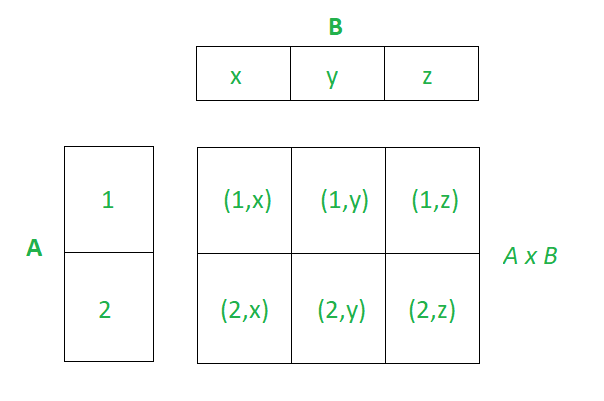Related Articles

# Cartesian Product of Sets

• Difficulty Level : Easy
• Last Updated : 25 May, 2021

The term ‘product‘ mathematically signifies the result obtained when two or more values are multiplied together. For example, 45 is the product of 9 and 5. One must be familiar with the basic operations on sets like Union and Intersection, which are performed on 2 or more sets. Cartesian Product is also one such operation that is performed on two sets, which returns a set of ordered pairs.

### What is an Ordered Pair?

An ordered pair is a pair of objects where one element is assigned first, and the other element is assigned second, denoted by (a,b). Here ‘a’ is called the first component, and ‘b’ is called the second component of the ordered set.

Example: (5, 7) is an ordered pair of integers.

Note: (5, 7) ≠ (7, 5), an ordered pair (a, b) is equal to (x, y) only if a = x and b = y.

### Cartesian Product of Sets

A cartesian product of two non-empty sets A and B is the set of all possible ordered pairs where the first component of the pair is from A, and the second component of the pair is from B. The set of ordered pairs thus obtained is denoted by A×B.

A × B = {(a, b) : a ∈ A and b ∈ B}

Example:

Let A = {1, 2} and B = {4, 5, 6}

A × B = {(1, 4), (1, 5), (1, 6), (2, 4), (2, 5), (2, 6)}

Here the first component of every ordered pair is from set A the second component is from set B.

The Cartesian Product of two sets can be easily represented in the form of a matrix where both sets are on either axis, as shown in the image below. Cartesian Product of  A = {1,  2} and B = {x, y, z}### Properties of Cartesian Product

1. The Cartesian Product is non-commutative: A × B ≠ B × A

Example:

A = {1, 2} , B = {a, b}

A × B = {(1, a), (1, b), (2, a), (2, b)}

B × A = {(a, 1), (b, 1), (b, 1), (b, 2)}

Therefore as A ≠ B we have A × B ≠ B × A

2. A × B = B × A, only if A = B

Proof:

Let A × B = B × A then we have

A ⊆  B  and B ⊆  A, it follows that A = B

3. The cardinality of the Cartesian Product is defined as the number of elements in A × B and is equal to the product of cardinality of both sets: |A × B| = |A| * |B|

Proof:

Let a ∈ A then the number of ordered pair (a, b) such that b ∈ B is |B|.

Therefore we have have |B| choices for b for each a where a ∈ A therefore the number of element in A×B is |A| * |B|.

4. A × B = {∅}, if either A = {∅} or B = {∅}

Proof:

We know |{∅}| = 0.

Now we have |A × B| = |{∅}| = 0

As |A × B| = |A| * |B| , we get |A| * |B| = 0

Thus atleast one of |A| or |B| should be equal to 0

Hence either A = {∅} or B = {∅}

### Sample Problems on Ordered Pairs and Cartesian Product of Sets

Problem 1: Find the value of x and y given (2x – y,  25) = (15,  2x + y)?

Solution:

As we know from the property of ordered pairs, 2x – y = 15 and 25 = 2x + y.

Solving the linear equations we have x = 10 and y = 5.

Problem 2. Given A = {2, 3, 4 , 5} and B = {4 , 16 , 23}, a ∈ A, b ∈ B, find the set of ordered pairs such that a2 < b?

Solution:

As 22 < 16 and 23, 32 < 16 and 23, 42 < 23

We have the set of ordered pairs such that a2 < b is {(2, 16), (2, 23), (3, 16), (2, 23), (4, 23)}

Problem 3. If A = {9, 10} and B = {3, 4, 6}, find A × B and |A × B|?

Solution:

A × B = {(9, 3), (9, 4), (9, 6), (10, 3), (10, 4), (10, 6)}

|A × B| = |A| * |B| = 2 * 3 = 6

Problem 4. If A × B = {(a, x), (a, y ), (b, x ), (b, y)}, find A and B?

Solution:

We know A is the set of all first components in ordered pairs of A × B and

B is the set of the second component in the ordered pair of A × B.

Therefore A = {a, b} and B = {x, y}

Problem 5. Given A × B has 15 ordered pairs and A has 5 elements, find the number of elements in B?

Solution:

We know |A × B| = |A| * |B|, 15 = 5 * |B|

Therefore B has 15 / 5 = 3 elements.

Attention reader! Don’t stop learning now. Join the First-Step-to-DSA Course for Class 9 to 12 students , specifically designed to introduce data structures and algorithms to the class 9 to 12 students

My Personal Notes arrow_drop_up